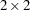Overview: SURVEYFREQ Procedure

The SURVEYFREQ procedure produces one-way to n-way frequency and crosstabulation tables from sample survey data. These tables include estimates of population totals, population proportions, and their standard errors. Confidence limits, coefficients of variation, and design effects are also available. The procedure provides a variety of options to customize the table display.

For one-way frequency tables, PROC SURVEYFREQ provides Rao-Scott chi-square goodness-of-fit tests, which are adjusted for the sample design. You can test a null hypothesis of equal proportions for a one-way frequency table, or you can input custom null hypothesis proportions for the test. For two-way tables, PROC SURVEYFREQ provides design-adjusted tests of independence, or no association, between the row and column variables. These tests include the Rao-Scott chi-square test, the Rao-Scott likelihood ratio test, the Wald chi-square test, and the Wald log-linear chi-square test. Fortables, PROC SURVEYFREQ computes estimates and confidence limits for risks (row proportions), the risk difference, the odds ratio, and relative risks.

PROC SURVEYFREQ computes variance estimates based on the sample design used to obtain the survey data. The design can be a complex multistage survey design with stratification, clustering, and unequal weighting. PROC SURVEYFREQ provides a choice of variance estimation methods, which include Taylor series linearization, balanced repeated replication (BRR), and the jackknife.

PROC SURVEYFREQ uses ODS Graphics to create graphs as part of its output. For general information about ODS Graphics, see Chapter 21, Statistical Graphics Using ODS. For specific information about the statistical graphics available with the SURVEYFREQ procedure, see the PLOTS= option in the TABLES statement and the section ODS Graphics.